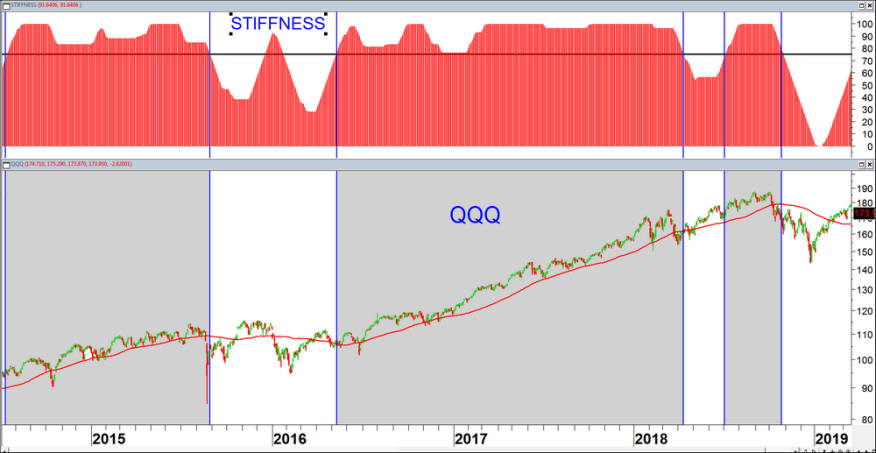This indicator was first introduced in my November 2018 article in Technical Analysis of STOCKS & COMMODITIES.

It  basically attempts to recognize strong price trends by counting the number of times that price was above the 100 day the moving average during the indicator period. The premise is that the less times that price penetrates the moving average, the stronger the trend is, suggesting less erratic prices in the future as well. I also introduced a volatility threshold that excludes minimal penetrations of less than 0.2 standard deviations.

The default moving average is 100 days and the indicator period is 60 days. In the example of QQQ below strong trend periods when the indicator is over 75 are shaded in grey.Daily chart of the QQQ ETF with the Stiffness (100,60) Indicator in the top window.Periods where the indicator is over 75 are shaded in light grey.

Amibroker Code:

//STIFFNESS INDICATOR
//Copyright Markos Katsanos 2018
Period = Param(“Stiffness Period”, 60, 40, 100, 10 );
MAB=Param(“Moving Average Period”,100,50,150,10);
SM=Param(“Smooth Coeff.”,2,1,4,1);
STIFFCRIT=Param(“STIFFCritical”,95,90,100,5);
NSTD=Param(“Min SD”,.5,0,1,.1);
//STIFFNESS
MA2=MA(C,MAB)-NSTD*StDev(C,MAB);
CLMA=C>MA2;
PENS=Sum(CLMA,PERIOD);
STIF=PENS*100/PERIOD; STIFFNESS=EMA(STIF,SM);
Plot(stiffness,”STIFFNESS”,colorRed ,styleHistogram );
Plot(STIFFCRIT,”STIFFCritical”,colorGreen,styleThick);